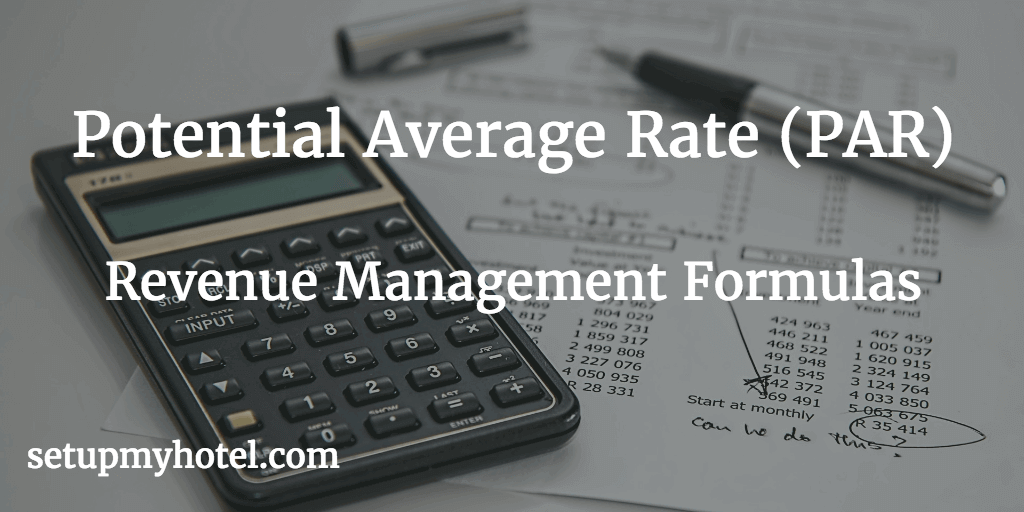# Potential Average Rate (PAR) Online Calculator

PAR or Potential Average Rate is a very important ratio in the revenue management department. The Potential Avg. Rate (PAR) is a collective statistics that combines the potential average rate, multiple occupancy percentages and the rate spread.

There are two steps involved to calculate the potential average rate:

Step 1: Is by multiplying the Rate Spread by the hotel's Multiple Occupancy Percentage.

Step 2: Add the result from Step 1 to hotel's Average Single Rate which will then give the 'Potential Average Rate (PAR)'

The Formula for Potential Average Rate (PAR)

Potential Average Single Rate = (Multiple Occupancy Percentage X Rate Spread) + Potential Average Single Rate

Potential Average Rate (PAR) Calculator:

Multiple Occ. %:

Potential Avg. Single Rate:

Potential Average Rate (PAR) =

Example 1 - Potential Avg. Rate:

Potential Avg. Single Rate         = 125.00

Potential Average Rate (PAR)    = (9.00 X 25.00) + 125

= (225) + 125

= 350

Example 2 - Potential Avg. Rate:

Multiple Occupancy Percentage = 5.00

Potential Avg. Single Rate         = 105.00

Potential Average Rate (PAR)    = (5.00 X 15.00) + 105.00

= (75) + 105

= 180